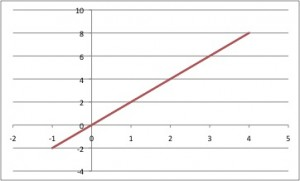# Math Help: Direct Variation

08/14/2015

## Algebra Help: Direct Variation

When the ratio between two variables always remains the same, the two variables are in direct variation. If A is always two times B, or the cost of apples is \$2 per pound, they directly vary. If I buy four pounds of apples, I pay \$8, and this relationship does not change. This article will discuss how to express and interpret direct variation.

### Direct Variation in an Equation

If one variable, y, is always two times another, x, it is expressed in the following equation: y/x=2 or y=2x. If y/x=1/3, y=1/3x, meaning that y is always three times smaller than x.

### Algebraic Interpretation

When we see an equation in the form of y=kx, we know that multiplying x by an amount also increases y by the same amount. For example, if k=2, and x=3, then y=6. If we multiply x*2 so that x=6, we also multiply y*2 and find that y=12.

### Geometric Interpretation

If we think of a square with sides of length x, and we know the equation for the perimeter (y) of a square is y=4x, we have an example of direct variation. If we double the length of a side, x, we also double the perimeter.

### Graphing Direct Variation

Equations in the form of y=kx are unique cases of the slope-intercept equation y=mx+b where the y-intercept (b) is 0 and m is the constant of variation. Lines of direct variation always pass through the origin (0,0) and have a slope called the constant of the variation. See the graph of our example, y=2x, below. In this case, the constant or slope is 2, because the ratio of these two variables is constantly two. If a line passes through the origin, you know that the two variables directly vary.## For Tutoring in West Caldwell, NJ

Call The Tutoring Center in West Caldwell at 862-210-8600 to schedule a free diagnostic assessment today. We offer one-to-one tutoring help in math, writing, reading, and test preparation. Find out more about our year-long academic programs online.

### FREE CONSULTATION

Schedule your Free Diagnostic Assessment Today!
Learn more about
THE TUTORING CENTER
on the national website: